# DDMRP calculationPosted by

Now it’s time ! After introducting DDMRP and Inventory positioning, followed by Buffer Level Definition and Calculation, it’s time to run the DDMRP after having understood and use the previous buffers and inventory positioning.

How it works ? Well, basically, nothing changes regarding the run of Master Plan. It’s exactly the same from a functional setup. The DDMRP will apply only for items with DDMRP item coverages, but the Master Plan remains the same as previous and so does the way of running it. Of course, it’s based on Planning Optimization meaning it’s run out of Dynamics 365 with high enhanced performances.

DDMRP suppose to run every days. Actually, the trigger for generating a planned order is set when the Net Flow is below the Reorder Point for each DDMRP item coverage.

Remainder of the Net Flow calculation : Net Flow = On hand + On order (Purchase orders, Production Orders, Transfer Orders) – Qualified demand

Regarding Qualified demand = Demand past due + Today’s demand (Sales orders) + Qualified order spikes

Let’s focus on Order Spikes. The Order spike threshold is a setup available on item coverage. It indicates the point where orders are not “normal” and qualified as extra orders.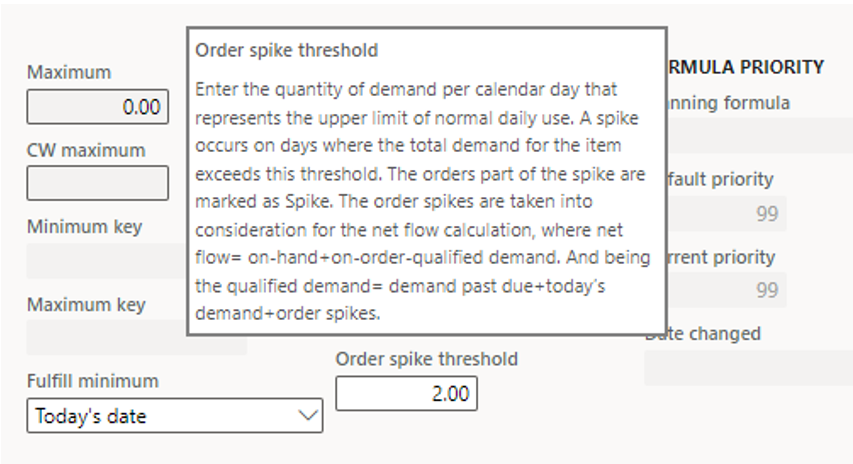To illustrate this, with an Average Daily Usage of 8 units (based on a average of 1 order), we can define that orders upper than 15 units are not normal. In such situation we can setup the Order spike threshold to 15.

That said, the DDMRP is able to take into account the past due (demand not fit), plus the order of today (that’s why the DDMRP is supposed to run every day), plus the extra orders called Qualified order spikes, within the time fence of course.

In other words, the system will not consider the sales order with a requirement date setup at tomorrow for generating a planned order (if < order spike threshold).

The quantity ordered follow this formula = Maximum – Net Flow.

The usage of Priority (see post before) instead of requirement dates is also calculated, and could be used for firming orders.

The next screenshot illustrates an example with a Net Flow at 147. In that case, DDMRP will generate a planned order of 130.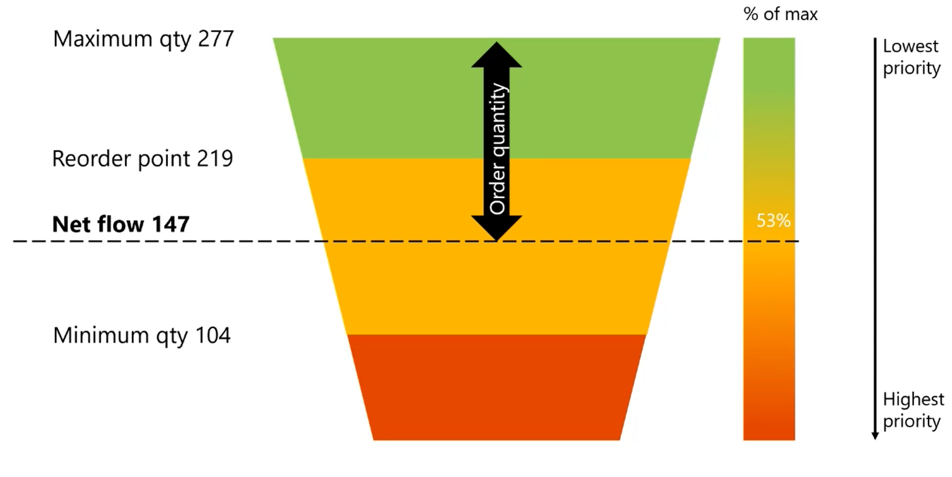Source : Tech Talk

Let’s have a look at an example.

## Use case 1

Here is my On-hand for SF1 = 95.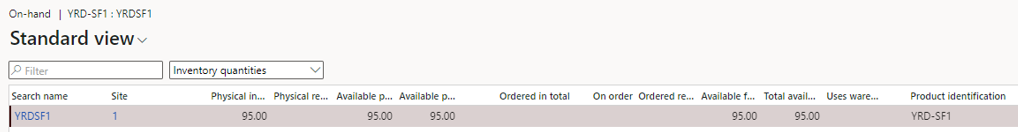I’ve already run the previous calculation mandatory for DDMRP (Average Daily Usage, Decoupling lead times, Minimum, Maximum and Reorder point).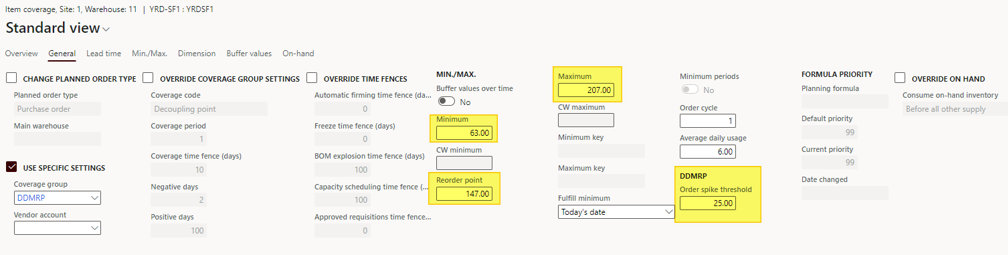The situation is :

• On-hand = 95
• Minimum = 63 ; Reorder point = 147; Maximum = 207
• Order spike threshold = 25
• On Order = 0
• Qualified demand = Demand past due + Today’s demand + Qualified order spikes = 0 + (today = 20/03) 0 + 0 = 0
• Net Flow = On-hand + On Order – Qualified demand = 95 + 0 – 0 = 95

Net flow < Reorder point, so DDMRP should generate a planned purchase order of : Maximum – Net Flow = 207 – 95 = 112

By running a Net Requirement update from the item, here is the result for the planned order.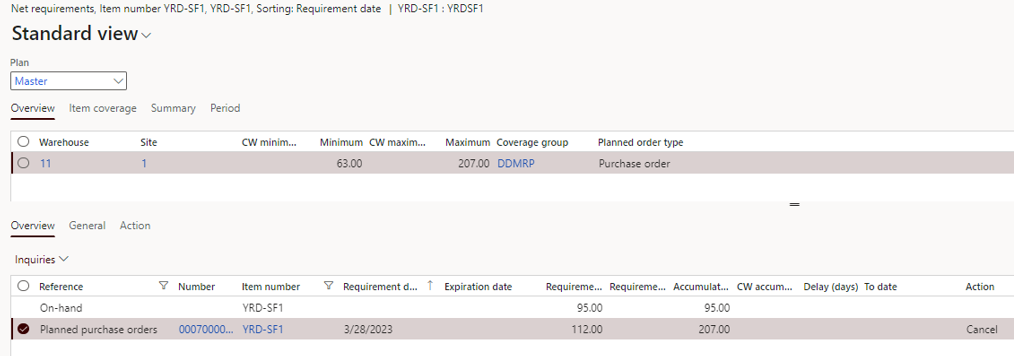## Use case 2

I’m adding On Order value with an estimated production order, for a quantity of 10.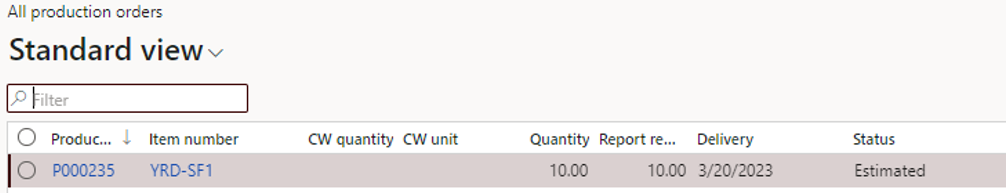The situation is :

• On-hand = 95
• Minimum = 63 ; Reorder point = 147; Maximum = 207
• Order spike threshold = 25
• On Order = 10
• Qualified demand = Demand past due + Today’s demand + Qualified order spikes = 0 + (today = 20/03) 0 + 0 = 0
• Net Flow = On-hand + On Order – Qualified demand = 95 + 10 – 0 = 105

Net flow < Reorder point, so DDMRP should generate a planned purchase order of : Maximum – Net Flow = 207 – 105 = 102

By running a Net Requirement update from the item, here is the result for the planned order.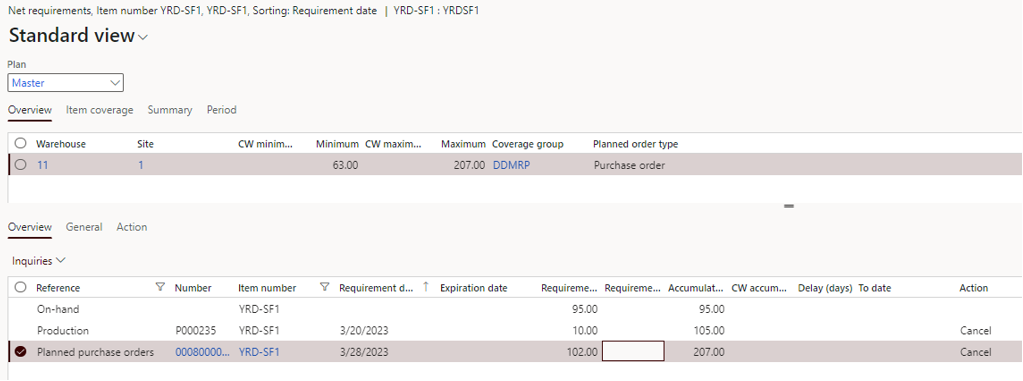Pay attention, the « On Order » from the DDMRP calculation is not the same as the « On Order » from the Inventory view.

In the first case, it take into account existing purchase orders, production and transfer orders.

In the Inventory view, it’s from the sales perspective. It could be confusing.

As you can see below, the 10 for the Production order are visible in the  « Ordered in total » column.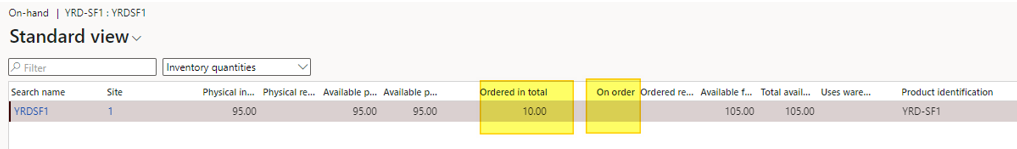## Use case 3

Pay attention here, only the sales orders are taken into account, even if you have setup forecast and if the Forecast model is setup as it should in the Master Plan.

See the Demand forecast of 20 :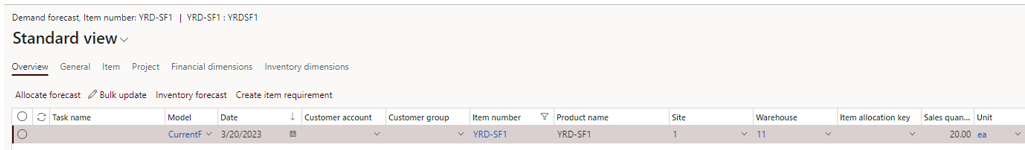See the sales order line of 5 for today.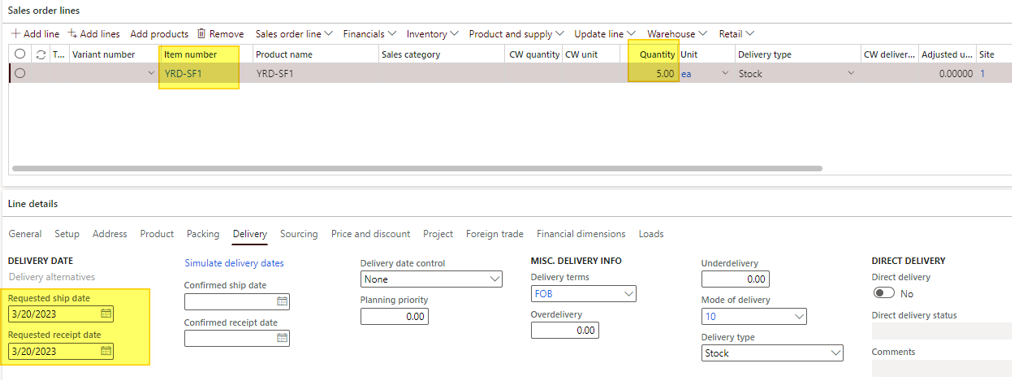The situation is :

• On-hand = 95
• Minimum = 63 ; Reorder point = 147; Maximum = 207
• Order spike threshold = 25
• On Order = 10
• Qualified demand = Demand past due + Today’s demand + Qualified order spikes = 0 + 5 + 0= 5
• Net Flow = On-hand + On Order – Qualified demand = 95 + 10 – 5 = 100

Net flow < Reorder point, so DDMRP should generate a planned purchase order of : Maximum – Net Flow = 207 – 100 = 107

By running a Net Requirement update from the item, here is the result for the planned order.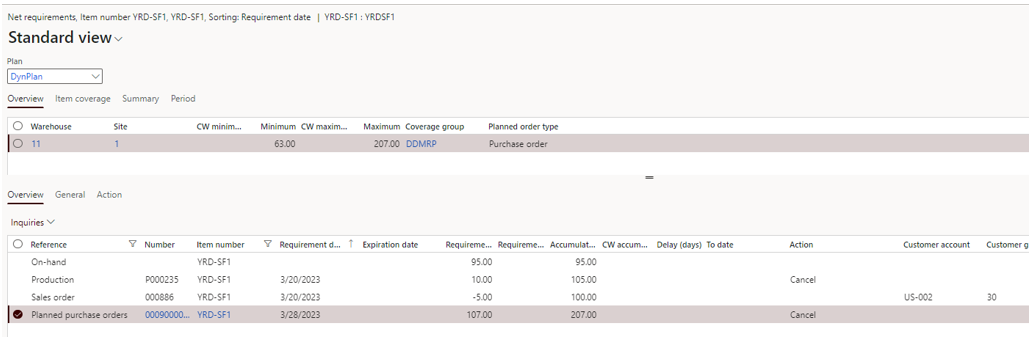## Use case 4 : with order spike

Now I’m adding a sales order, with a requested ship date far in the future, for a quantity of 30.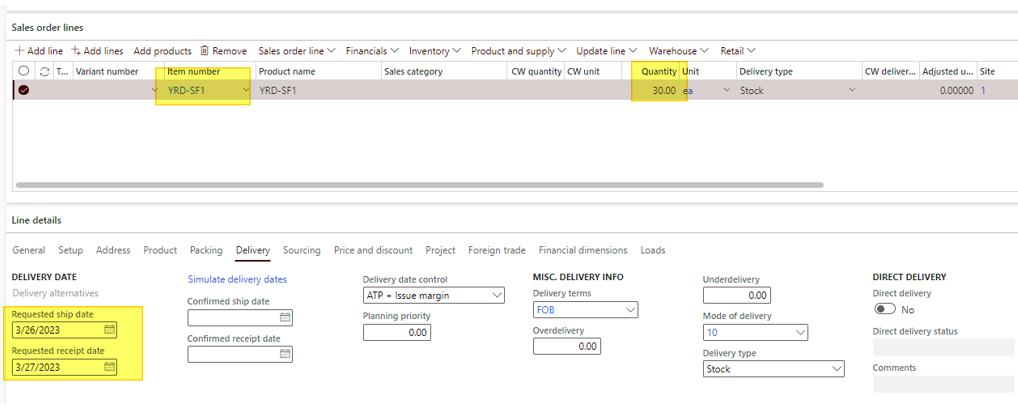The situation is :

• On-hand = 95
• Minimum = 63 ; Reorder point = 147; Maximum = 207
• Order spike threshold = 25
• On Order = 10
• Qualified demand = Demand past due + Today’s demand + Qualified order spikes = 0 + 5 + 30 = 35
• Net Flow = On-hand + On Order – Qualified demand = 95 + 10 – 35 = 70

Net flow < Reorder point, so DDMRP should generate a planned purchase order of : Maximum – Net Flow = 207 – 70 = 137

By running a Net Requirement update from the item, here is the result for the planned order.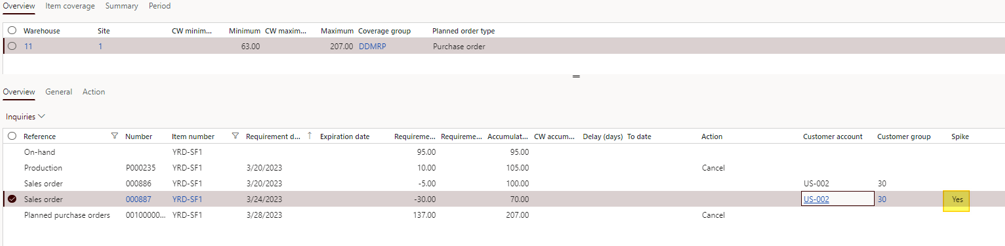As you can see, the sales order is flagged as a Spike order.

That’s it for the tests of DDMRP calculation.

Yohann

Ce site utilise Akismet pour réduire les indésirables. En savoir plus sur comment les données de vos commentaires sont utilisées.